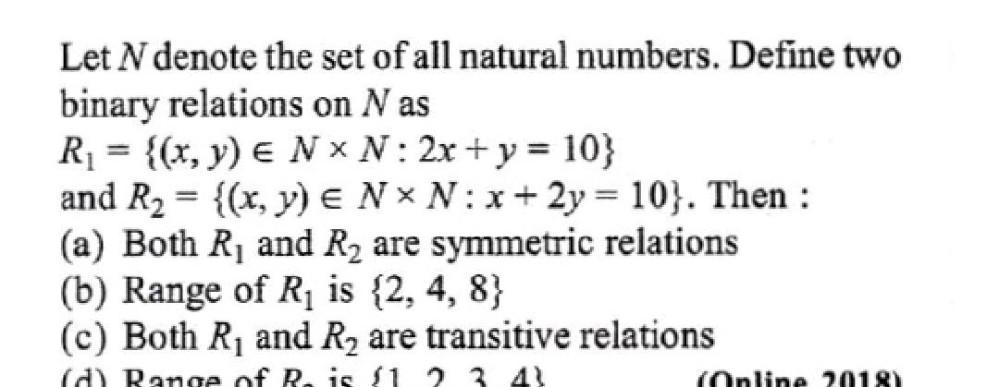Question:

# Let N denote the set of all natural numbers. Define two binary relations on N as R₁ = {(x, y) ∈ N × N: 2x + y = 10} and R₂ = {(x, y) ∈ N × N: x + 2y = 10}. Then : (a) Both R₁ and R₂ are symmetric relLet N denote the set of all natural numbers. Define two binary relations on N as R₁ = {(x, y) ∈ N × N: 2x + y = 10} and R₂ = {(x, y) ∈ N × N: x + 2y = 10}. Then : (a) Both R₁ and R₂ are symmetric relations (b) Range of R₁ is {2, 4, 8} (c) Both R₁ and R₂ are transitive relations (d) Range of R₂ is {1,2,3,4}